A quadratic function is a 2nd-degree polynomial function: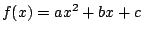, where.

The graph of a quadratic function is a parabola, which opens up ifand opens down if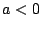.

The x-coordinate of the vertex of the parabola is given by, and the y-coordinate can be found by substituting this value forinto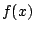.

If the vertex of the parabola has coordinates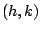, then the standard equation of the parabola has the form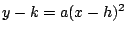.

The x-intercepts of the parabola, if there are any, are the solutions of the quadratic equation.

Ex 1 Find the vertex of the parabola.

Sol We have that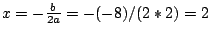, and then.

Ex 2 Find the minimum value of the function.

Sol The minimum value of this function is given by the y-coordinate of the vertex. Since the x-coordinate of the vertex is given by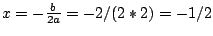, the minimum value is given by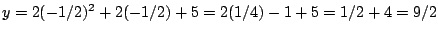.

Pr 1 Find the vertex of the parabola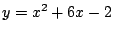.

Pr 2 Find the maximum value of the function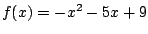.

Pr 3 Find a quadratic functionwhich has 5 and 1 as the x-intercepts of its graph and which has a minimum value of -12.

Pr 4 Find a parabolawhich has its vertex at the pointand which passes through the point.

Pr 5 Find the minimum value of the function, and find the values offor which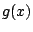is a minimum.

Pr 6 Find the vertex of the parabola.

Pr 7 Find a quadratic functionsuch that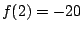is its minimum value, and such that.

Pr 8 Find an equation of the non-vertical line which intersects the parabolaonly at the point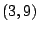.

Pr 9 Find the maximum value for the function.